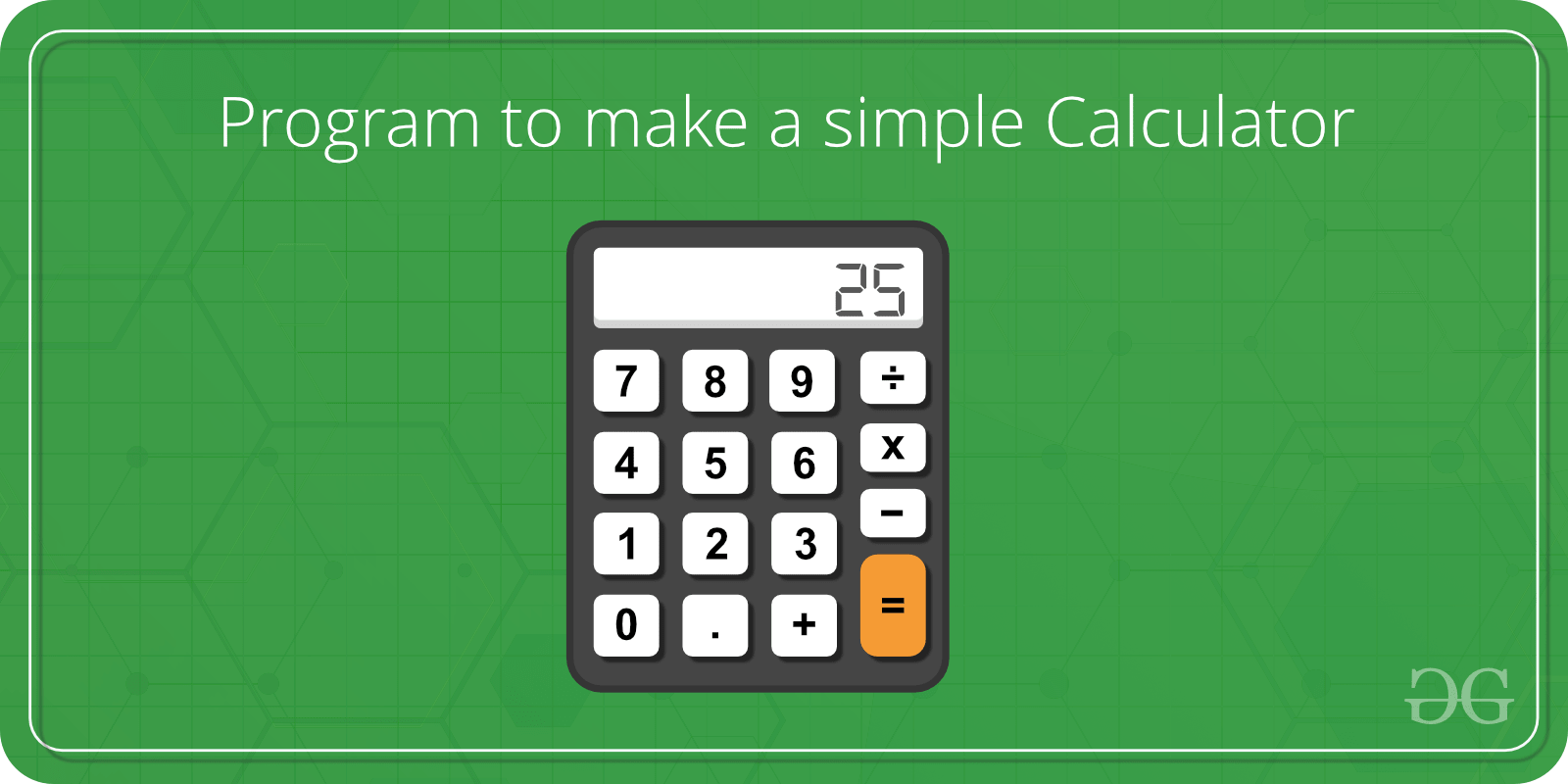Related Articles
Menu Driven C++ Program for a Simple Calculator
• Difficulty Level : Easy
• Last Updated : 16 Jul, 2019

Problem Statement:
Write a menu-driven program using the Switch case to calculate the following:

2. Difference between two numbers
3. Product of two numbers
4. Division of two numbers
5. HCF of two numbers
6. LCM of two numbersExamples:

```Input: num1 = 5, num2 = 7, choice = 1
Output: Sum is 12

Input: num1 = 5, num2 = 7, choice = 5
Output: GCD is 1
```

## Recommended: Please try your approach on {IDE} first, before moving on to the solution.

Implementation:

 `// C++ program to illustrate``// Menu-Driven program using Switch-case`` ` `#include ``using` `namespace` `std;`` ` `// Function to display the menu``void` `menu()``{``    ``cout << ``"Press 1 to calculate Sum of Numbers\n"``;``    ``cout << ``"Press 2 to calculate Difference of Numbers\n"``;``    ``cout << ``"Press 3 to calculate Product of numbers\n"``;``    ``cout << ``"Press 4 to calculate Division of numbers\n"``;``    ``cout << ``"Press 5 to calculate HCF of numbers\n"``;``    ``cout << ``"Press 6 to calculate LCM of numbers\n"``;``    ``cout << ``"Press 7 to exit\n"``;``}`` ` `// Function to calculate and display the result``void` `result(``int` `choice, ``int` `a, ``int` `b)``{`` ` `    ``// Display the result``    ``switch` `(choice) {``    ``case` `1: {``        ``cout << ``"Sum is "` `<< (a + b) << ``"\n"``;``        ``break``;``    ``}``    ``case` `2: {``        ``cout << ``"Difference is "` `<< (a - b) << ``"\n"``;``        ``break``;``    ``}``    ``case` `3: {``        ``cout << ``"Product is "` `<< (a * b) << ``"\n"``;``        ``break``;``    ``}``    ``case` `4: {``        ``cout << ``"Division is "` `<< (a / b) << ``"\n"``;``        ``break``;``    ``}``    ``case` `5: {``        ``cout << ``"GCD is "` `<< __gcd(a, b) << ``"\n"``;``        ``break``;``    ``}``    ``case` `6: {``        ``cout << ``"LCM is "``             ``<< ((a * b) / __gcd(a, b))``             ``<< ``"\n"``;``        ``break``;``    ``}``    ``case` `7: {``        ``cout << ``"Thank you\n"``;``        ``break``;``    ``}``    ``default``:``        ``printf``(``"Wrong Input\n"``);``    ``}``}`` ` `int` `main()``{`` ` `    ``// Get the two numbers``    ``int` `a = 5, b = 7;`` ` `    ``int` `choice, res;`` ` `    ``// Display the menu``    ``menu();`` ` `    ``// Enter the choice``    ``cout << ``"Enter your choice:\n"``;``    ``choice = 1;``    ``cout << ``"Choice is "` `<< choice << endl;`` ` `    ``// Display the result``    ``// according to the choice``    ``result(choice, a, b);`` ` `    ``return` `0;``}`
Output:
```Press 1 to calculate Sum of Numbers
Press 2 to calculate Difference of Numbers
Press 3 to calculate Product of numbers
Press 4 to calculate Division of numbers
Press 5 to calculate HCF of numbers
Press 6 to calculate LCM of numbers
Press 7 to exit